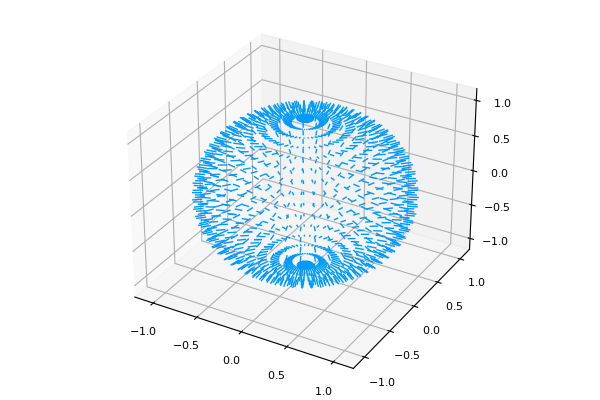# 3d quiverusing Plots
pyplot()
ϕs = range(-π, π, length = 50)
θs = range(0, π, length = 25)
θqs = range(1, π - 1, length = 25)
x = vec([sin(θ) * cos(ϕ) for (ϕ, θ) = Iterators.product(ϕs, θs)])
y = vec([sin(θ) * sin(ϕ) for (ϕ, θ) = Iterators.product(ϕs, θs)])
z = vec([cos(θ) for (ϕ, θ) = Iterators.product(ϕs, θs)])
u = 0.1 * vec([sin(θ) * cos(ϕ) for (ϕ, θ) = Iterators.product(ϕs, θqs)])
v = 0.1 * vec([sin(θ) * sin(ϕ) for (ϕ, θ) = Iterators.product(ϕs, θqs)])
w = 0.1 * vec([cos(θ) for (ϕ, θ) = Iterators.product(ϕs, θqs)])
quiver(x, y, z, quiver = (u, v, w))